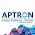## What is Confusion Matrix and Advanced Classification Metrics?

After data preparation and model training, there is model evaluation phase which I mentioned in my earlier article Simple Picture of Machine Learning Modelling Process

Once model is developed, the next phase is to calculate the performance of the developed model using some evaluation metrics. In this article, you will just discover about confusion matrix though there are many classification metrics out there.

Mainly, it focuses on below points:

• What is confusion matrix?
• Four outputs in confusion matrix

Table 1. Confusion matrix with advanced classification metrics

Confusion Matrix is a tool to determine the performance of classifier. It contains information about actual and predicted classifications. The below table shows confusion matrix of two-class, spam and non-spam classifier.

Table 2. Confusion matrix of email classification

Let’s understand four outputs in confusion matrix.

1. True Positive (TP) is the number of correct predictions that an example is positive which means positive class correctly identified as positive.
Example: Given class is spam and the classifier has been correctly predicted it as spam.

2. False Negative (FN) is the number of incorrect predictions that an example is negative which means positive class incorrectly identified as negative.
Example: Given class is spam however, the classifier has been incorrectly predicted it as non-spam.

3. False positive (FP) is the number of incorrect predictions that an example is positive which means negative class incorrectly identified as positive.
Example: Given class is non-spam however, the classifier has been incorrectly predicted it as spam.

4. True Negative (TN) is the number of correct predictions that an example is negative which means negative class correctly identified as negative.
Example: Given class is spam and the classifier has been correctly predicted it as negative.

Now, let’s see some advanced classification metrics based on confusion matrix. These metrics are mathematically expressed in Table 1 with example of email classification, shown in Table 2. Classification problem has spam and non-spam classes and dataset contains 100 examples, 65 are Spams and 35 are non-spams.

Sensitivity is also referred as True Positive Rate or Recall. It is measure of positive examples labeled as positive by classifier. It should be higher. For instance, proportion of emails which are spam among all spam emails.

Table 3. Sensitivity in confusion matrix

Sensitivity = 45/(45+20) = 69.23% .

The 69.23% spam emails are correctly classified and excluded from all non-spam emails.

Specificity is also know as True Negative Rate. It is measure of negative examples labeled as negative by classifier. There should be high specificity. For instance, proportion of emails which are non-spam among all non-spam emails.

Table 4. Specificity in confusion matrix

specificity = 30/(30+5) = 85.71% .

The 85.71% non-spam emails are accurately classified and excluded from all spam emails.

Precision is ratio of total number of correctly classified positive examples and the total number of predicted positive examples. It shows correctness achieved in positive prediction.

Table 5. Precision in confusion matrix

Precision = 45/(45+5)= 90%

The 90% of examples are classified as spam are actually spam.

Accuracy is the proportion of the total number of predictions that are correct.

Table 6. Accuracy in confusion matrix

Accuracy = (45+30)/(45+20+5+30) = 75%

The 75% of examples are correctly classified by the classifier.

F1 score is a weighted average of the recall (sensitivity) and precision. F1 score might be good choice when you seek to balance between Precision and Recall.

It helps to compute recall and precision in one equation so that the problem to distinguish the models with low recall and high precision or vice versa could be solved.

Kindly follow my blog by email and stay tuned for more advanced post on regression measures.

Thank you!

1.Great,thanku :)

1.2.Good work! Please post some more articles.

1.3.Measures are clearly explained with great addition of figures. Thanks for sharing!

1.Thanks Sanjay!

4.Good note...Appreciate for sharing

1.5.Good work! Thank you.

6.Nice explanation

7.Thank you. Well explained.

8.beautifully explained. Manisha could you please share your official email address on your profile. I want to discuss one opportunity with you regarding deep learning education.

9.It is amazing and wonderful to visit your site.Thanks for sharing this information,this is useful to me...
http://chennaitraining.in/building-estimation-and-costing-training-in-chennai/
http://chennaitraining.in/embedded-systems-training-in-chennai/
http://chennaitraining.in/vlsi-training-in-chennai/
http://chennaitraining.in/matlab-training-in-chennai/
http://chennaitraining.in/iot-training-in-chennai/

10.My spouse and I love your blog and find almost all of your posts to be just what I’m looking for. Appreciating the persistence you put into your blog and the detailed information you provide. I found another one blog like you Data Analytics .Actually I was looking for the same information on internet for Data Analytics Service and came across your blog. I am impressed by the information that you have on this blog. Thanks once more for all the details.

11.Thanks for sharing this Information. Data Science Institute in Gurgaon